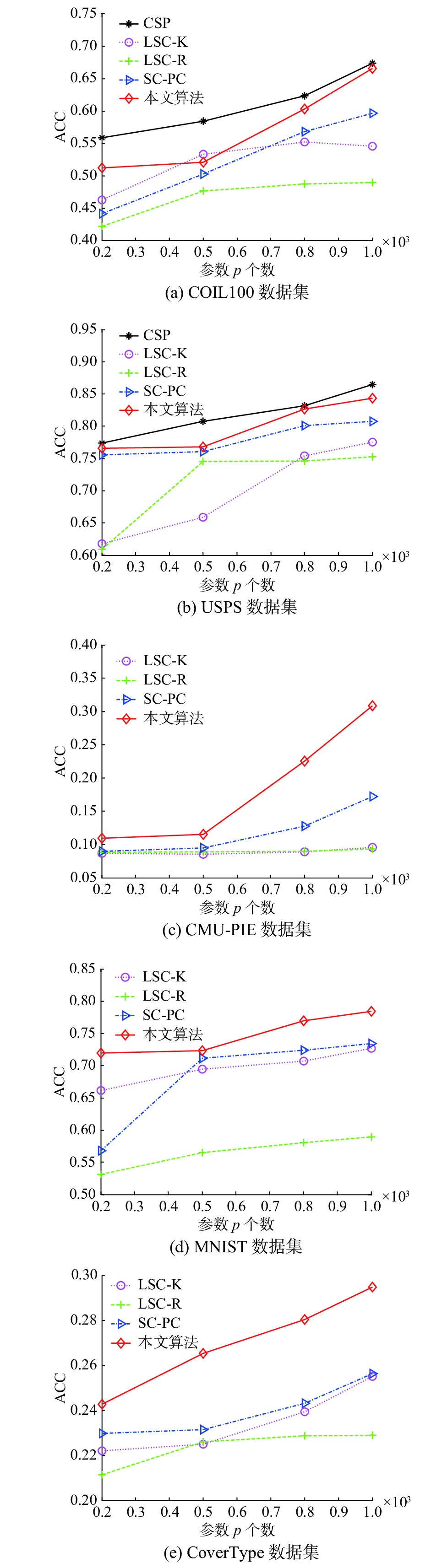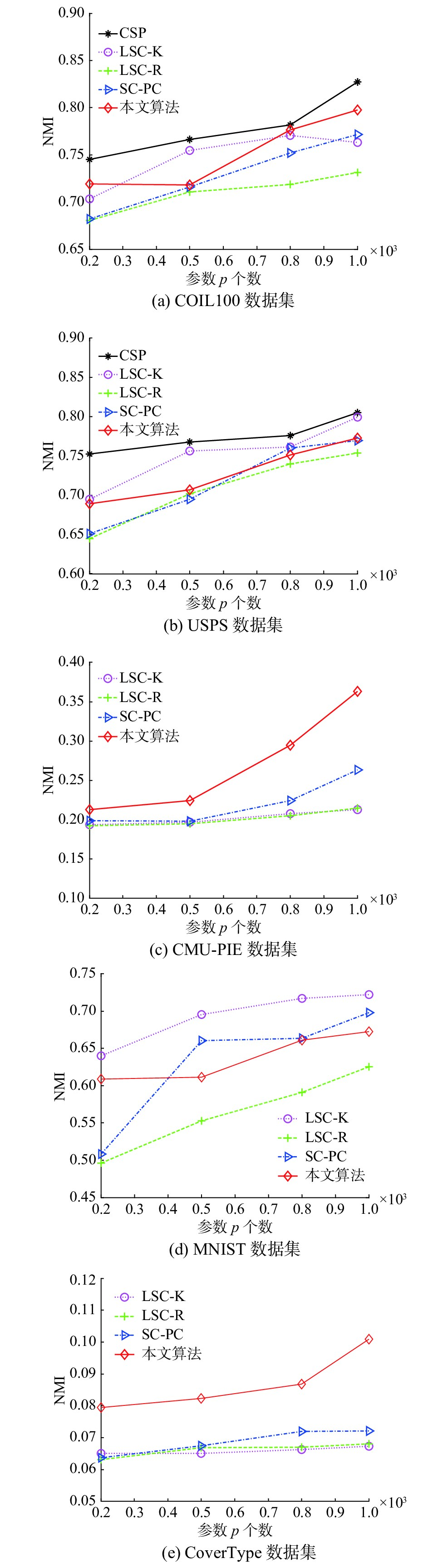﻿ 结合稀疏表示与约束传递的半监督谱聚类算法
«上一篇文章快速检索 高级检索

 智能系统学报2018, Vol. 13Issue (5): 855-863  DOI: 10.11992/tis.2017030130

### 引用本文ZHAO Xiaoxiao, ZHOU Zhiping. A semi-supervised spectral clustering algorithm combined with sparse representation and constraint propagation[J]. CAAI Transactions on Intelligent Systems, 2018, 13(5): 855-863. DOI: 10.11992/tis.201703013.### 文章历史

A semi-supervised spectral clustering algorithm combined with sparse representation and constraint propagation
ZHAO Xiaoxiao, ZHOU Zhiping
Engineering Research Center of Internet of Things Technology Applications Ministry of Education, Jiangnan University, Wuxi 214122, China
Abstract: The semi-supervised spectral clustering algorithm does not deal with large-scale datasets effectively and does not fully utilize the constraint information because it does not consider the constraint propagation. To address these drawbacks, this paper proposes a semi-supervised spectral clustering algorithm that combines sparse representation and constraint propagation. The algorithm first generates the constraint matrix according to the constraint information, introduces it into the spectral clustering, and then constructs a sparse representation matrix by taking the data points in the constrained sets as the landmarks to approximate the graph similarity matrix, thereby revising the constrained spectral clustering model. Meanwhile, the connected region is generated according to the similarity matrix of the landmark data points, and the neighboring nodes are dynamically adjusted in each connected region. The clustering accuracy is further improved using the constraint propagation. Experimental results show that the proposed method is more efficient than constrained spectral clustering algorithms, and their accuracy levels are similar. Moreover, its clustering accuracy exceeds those of the fast spectral clustering algorithms.
Key words: data mining    cluster analysis    spectral clustering    semi-supervised learning    sparse representation    constraint propagation

1 算法基本原理 1.1 约束NCut算法

 $\mathop {\arg \min }\limits_{{{v}} \in {\mathbb{R}^n}} {{{v}}^{\rm{T}}}{{Lv}},{\rm{ }}\,{\rm{s}}{\rm{.t}}{\rm{. }}{{{v}}^{\rm{T}}}{{v}} = {{1}},{{v}} \bot {{1}}$ (1)

 {{{Q}}_{ij}}{\rm{ = }}\left\{ {\begin{aligned}& 1,\quad {({{{x}}_i},{{{x}}_j}) \in {M}}\\&- 1,\quad {({{{x}}_i},{{{x}}_j}) \in {C}}\\& 0,\quad {{\text{无约束信息}}}\end{aligned}} \right. (2)

 ${{{v}}^{\rm{T}}}{{Qv}} = \sum\limits_{i = 1}^n {\sum\limits_{j = 1}^n {{{{v}}_i}{{{v}}_j}{Q_{ij}}} }$ (3)

 $\mathop {\arg \min }\limits_{{{v}} \in {^n}} {{{v}}^{\rm{T}}}{{Lv}},{\rm{ }}\,{\rm{s}}{\rm{.t}}{\rm{. }}{{{v}}^{\rm{T}}}{{Qv}} \geqslant \alpha ,{{{v}}^{\rm{T}}}{{v}} = {{1}},{{v}} \bot {{1}}$ (4)

1.2 依据稀疏表示的相似度矩阵

 ${{{\hat x}}_i} = \sum\limits_{j = 1}^p {{{Z}_{ji}}{{{y}}_j}}$ (5)

 ${Z_{ji}} = \frac{{{{K}}({{{x}}_i},{{{y}}_j})}}{{\sum\nolimits_{j' \in \left\langle i \right\rangle } {{{K}}({{{x}}_i},{{{y}}_{j'}})} }},\quad i \in 1,2, \cdots ,n, j \in \left\langle i \right\rangle$ (6)

2 所提算法 2.1 约束关系传递

 {{\hat Z}}\left( {{p_i},{p_j}} \right) = \left\{ {\begin{aligned}& {1,} \quad {\left\{ {{p_i} \in {T_a}|{p_j} \in {T_a}} \right\}} \\ & {0,} \quad {\left\{ {{p_i} \in {T_a}|{p_j} \in {{T_a^{\prime}}}} \right\}} \end{aligned}} \right. (7)

 {\bf{lookupVec}}_{i'} = \left\{ {\begin{aligned}& {\min + {\rm{fre}}{{\rm{q}}_{i'}} \times \displaystyle\frac{{\max - \min }}{{m - 1}},}\quad {m \ne 1} \\ & {\max ,}\quad {m = 1} \end{aligned}} \right. (8)

${{{L}}_{{p_i}}}$ ${{{L}}_{{p_j}}}$ 分别表示在同一个连通区域内的数据 ${p_i}$ ${p_j}$ r近邻集合， ${p_i},{p_j} \in {T_a}$ $i,j \in \left\{ {1,2, \cdots ,\left| {{T_a}} \right|} \right\}$ 。因为矩阵Z具有高度稀疏性，在同一个连通区域内的两个数据点 ${p_i}$ ${p_j}$ 可能并没有共同近邻点。所以可考虑对于 ${T_a}$ 内的任一数据点 ${p_i}$ ，将其他数据点 ${p_j} \in {T_a}$ 的近邻点也作为 ${p_i}$ 的近邻点：

 ${{{L}}_{{p_i}}} = \left\{ {{{{L}}_{{p_1}}} \cup {{{L}}_{{p_2}}} \cup \cdots \cup {{{L}}_{{p_{\left| a \right|}}}}} \right\}, \quad i \in 1,2, \cdots ,\left| {{T_a}} \right|$ (9)

 ${{\hat Z}}\left( {i,{p_j}} \right) = {\bf{lookupVec}}_{i'}$ (10)

2.2 依据稀疏表示的可扩展半监督NCut算法

 $\mathop {\arg \min }\limits_{v \in {\mathbb{R}^n}} {{{v}}^{\rm{T}}}{{\bar Lv}},{\rm{ }}\,\,{\rm{s}}{\rm{.t}}{\rm{. }}{{{v}}^{\rm{T}}}{{Qv}} \geqslant \alpha ,{{{v}}^{\rm{T}}}{{v}} = {{1}},{{v}} \bot {{1}}$ (11)

 ${{Lv}} = \lambda \left( {{{Q}} - \beta {{I}}} \right){{v}}$ (12)

 $\mathop {\arg \min }\limits_{{{u}} \in {\mathbb{R}^p}} {{{u}}^{\rm{T}}}{{Au}},{\rm{ }}\,{\rm{s}}{\rm{.t}}{\rm{. }}{{{u}}^{\rm{T}}}{{\hat Qu}} \geqslant \alpha ,{{{u}}^{\rm{T}}}{{\hat Su}} = {{1}},{{{1}}^{\rm{T}}}{{\hat Su}} = 0$ (13)

 $\Lambda \left( {{{u}},\lambda ,\mu } \right) = {{{u}}^{\rm{T}}}{{Au}} - \lambda \left( {{{{u}}^{\rm{T}}}{{\hat Qu}} - \alpha } \right) - \mu \left( {{{{u}}^{\rm{T}}}{{\hat Su - 1}}} \right)$ (14)

 ${{Au}} - \lambda {{\hat Qu}} - \mu {{\hat Su}} = 0$ (15)
 ${{{u}}^{\rm{T}}}{{\hat Qu}} \geqslant \alpha$
 ${{{u}}^{\rm{T}}}{{\hat Su}} = {{1}}$
 ${{{1}}^{\rm{T}}}{{\hat Su}} = 0$
 $\lambda \geqslant 0$
 $\lambda \left( {{{{u}}^{\rm{T}}}{{\hat Qu}} - \alpha } \right) = 0$

$\lambda = 0$ 时，式(15)变为 ${{Au}} - \mu {{\hat Su}} = 0$ ，意味着并没有考虑相关约束信息，所以为了充分使用约束信息，只考虑 $\lambda > 0$ 的情况，即 ${{{u}}^{\rm{T}}}{{\hat Qu}} = \alpha$

 ${{Au}} = \lambda \left( {{{\hat Q}} - \beta {{\hat S}}} \right){{u}}$ (16)

 $\lambda {{{u}}^{\rm{T}}}\left( {{{\hat Q}} - \beta {{\hat S}}} \right){{u}} = \lambda \left( {\alpha - \beta } \right) \geqslant 0$

1) 将在约束集合内的p个点选为地标点，并作为矩阵U的列向量；

2) 根据式(6)构造Z，并计算 ${{\hat Z = }}{{{D}}^{ - {1 / 2}}}{{Z}}$

3) 使用Tarjan算法，根据地标点之间的约束关系计算连通区域CC；

4) for 每个连通区域CC do：

end for

5) 依据约束关系生成约束矩阵 ${{Q}}$ ，计算 ${{\hat S = \hat Z}}{{{\hat Z}}^{\rm{T}}}$ ${{\hat Q = \hat ZQ}}{{{\hat Z}}^{\rm{T}}}$

6) 求解 ${{\hat Q}}x = \gamma {{\hat S}}x$ 的最大特征值 ${\gamma _{\max }}$

7) if $\;\beta \geqslant {\gamma _{\max }}$ ，类label的向量 ${{V}} =$ Ø；

8) else求解式(16)的特征向量u

9) 找出所有正特征值所对应的特征向量 $\left\{ {{{u}}_i^ + } \right\}$ $\left\{ {{{u}}_i^ + } \right\}$ 中每个元素乘 $\sqrt {\displaystyle\frac{1}{{{{{u}}_i}{{\hat S}}{{{u}}_i}}}}$

10) 去掉 $\left\{ {{{u}}_i^ + } \right\}$ 中与 ${{{1}}^{\rm{T}}}{{\hat S}}$ 不正交的特征向量；

11) 计算 ${{u}}_i^{\rm{T}}{{A}}{{{u}}_i}$ ，寻找m个特征向量使 ${{u}}_i^{\rm{T}}{{A}}{{{u}}_i}$ 最小， $m = \min \left\{ {k - 1,\left| {\left\{ {{{u}}_i^ + } \right\}} \right|} \right\}$ ，并把这些特征向量作为 ${{V}}$ 的列向量；

12) 计算 ${{{V}}^{(r)}} = {{{\hat Z}}^{\rm{T}}}{{V}}\left( {{{I - }}{{{V}}^{\rm{T}}}{{AV}}} \right)$ ，并对其进行k-means聚类。

2.3 算法复杂度分析

3 实验与分析 3.1 实验环境表 1 实验数据集的特性 Tab.1 Experimental datasets features

3.2 实验分析表 2 各数据集实验结果 Tab.2 Experimental results of different datasetsDownload: 图 1 不同算法的ACC比较 Fig. 1 Comparison of the ACCs of different algorithmsDownload: 图 2 不同算法的NMI比较 Fig. 2 Comparison of the NMIs of different algorithms

4 结束语

  VON Luxburg U. A tutorial on spectral clustering[J]. Statistics and computing, 2007, 17(4): 395-416. DOI:10.1007/s11222-007-9033-z (0)  SHI Jianbo, MALIK J. Normalized cuts and image segmentation[J]. IEEE transactions on pattern analysis and machine intelligence, 2000, 22(8): 888-905. DOI:10.1109/34.868688 (0)  HU Han, FENG Jianjiang, YU Chuan, et al. Multi-class constrained normalized cut with hard, soft, unary and pairwise priors and its applications to object segmentation[J]. IEEE transactions on image processing, 2013, 22(11): 4328-4340. DOI:10.1109/TIP.2013.2271865 (0)  刘建伟, 刘媛, 罗雄麟. 半监督学习方法[J]. 计算机学报, 2015, 38(8): 1592-1617. LIU Jianwei, LIU Yuan, LUO Xionglin. semi-supervised learning methods[J]. Chinese journal of computers, 2015, 38(8): 1592-1617. (0)  ALUSH A, FRIEDMAN A, Goldberger J. Pairwise clustering based on the mutual-information criterion[J]. Neurocomputing, 2016, 182: 284-293. DOI:10.1016/j.neucom.2015.12.025 (0)  FORESTIER G, WEMMERT C. Semi-supervised learning using multiple clusterings with limited labeled data[J]. Information sciences, 2016, 361-362: 48-65. DOI:10.1016/j.ins.2016.04.040 (0)  KAMVAR S D, KLEIN D, MANNING C D. Spectral learning[C]//Proceedings of the 18th International Joint Conference on Artificial Intelligence. Acapulco, Mexico, 2003: 561–566. (0)  蒋伟进, 许宇晖, 王欣. 基于谱图和成对约束的主动半监督聚类算法[J]. 控制与决策, 2013, 28(6): 904-908. JIANG Weijin, XU Yuhui, WANG Xin. Active semi-supervised clustering algorithm based-on pair-wise constraints[J]. Control and decision, 2013, 28(6): 904-908. (0)  DING Shifei, JIA Hongjie, ZHANG Liwen, et al. Research of semi-supervised spectral clustering algorithm based on pairwise constraints[J]. Neural computing and applications, 2014, 24(1): 211-219. DOI:10.1007/s00521-012-1207-8 (0)  CUCURINGU M, KOUTIS I, CHAWLA S, et al. Simple and scalable constrained clustering: a generalized spectral method[C]//Proceedings of the 19th International Conference on Artificial Intelligence and Statistics. Cadiz, Spain, 2016: 445–454. (0)  WANG Xiang, QIAN Buyue, DAVIDSON I. On constrained spectral clustering and its applications[J]. Data mining and knowledge discovery, 2014, 28(1): 1-30. DOI:10.1007/s10618-012-0291-9 (0)  YU Zhiwen, LUO Peinan, YOU J, et al. Incremental semi-supervised clustering ensemble for high dimensional data clustering[J]. IEEE transactions on knowledge and data engineering, 2016, 28(3): 701-714. DOI:10.1109/TKDE.2015.2499200 (0)  LU Zhiwu, PENG Yuxin. Exhaustive and efficient constraint propagation: a graph-based learning approach and its applications[J]. International journal of computer vision, 2013, 103(3): 306-325. DOI:10.1007/s11263-012-0602-z (0)  CAI Deng, CHEN Xinlei. Large scale spectral clustering via landmark-based sparse representation[J]. IEEE transactions on cybernetics, 2015, 45(8): 1669-1680. DOI:10.1109/TCYB.2014.2358564 (0)  SEMERTZIDIS T, RAFAILIDIS D, STRINTZIS M G, et al. Large-scale spectral clustering based on pairwise constraints[J]. Information processing and management, 2015, 51(5): 616-624. DOI:10.1016/j.ipm.2015.05.007 (0)